INTRODUCTION TO R
Erik Kusch
erik.kusch@i-solution.de
Section for Ecoinformatics & Biodiversity
Center for Biodiversity and Dynamics in a Changing World (BIOCHANGE)
Aarhus University
Aarhus University Biostatistics - Why? What? How? 1 / 42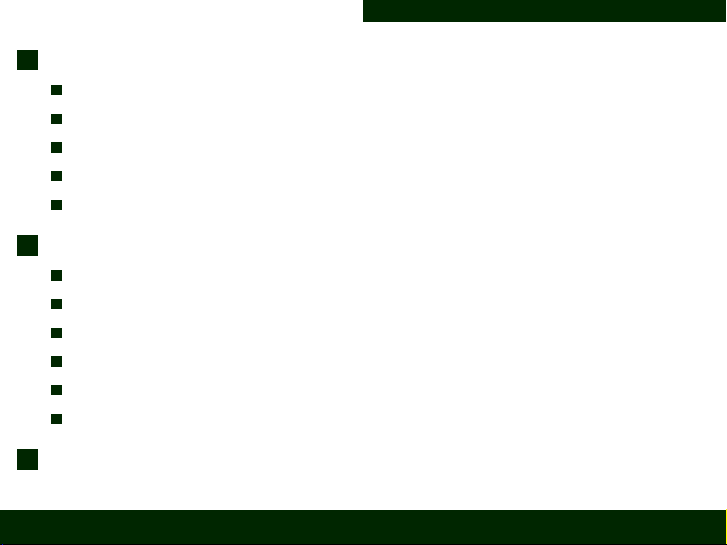1 Objects in R
The Basics
Basic R-internal Modes
Basic R-internal Types
CheatSheet Overview
Inspecting and Subsetting Objects in R
2 Functionality in R
Vectorisation
User-deﬁned Functions
Packages
Statements
Loops
Useful Commands In R
3 Exercise
Aarhus University Biostatistics - Why? What? How? 2 / 42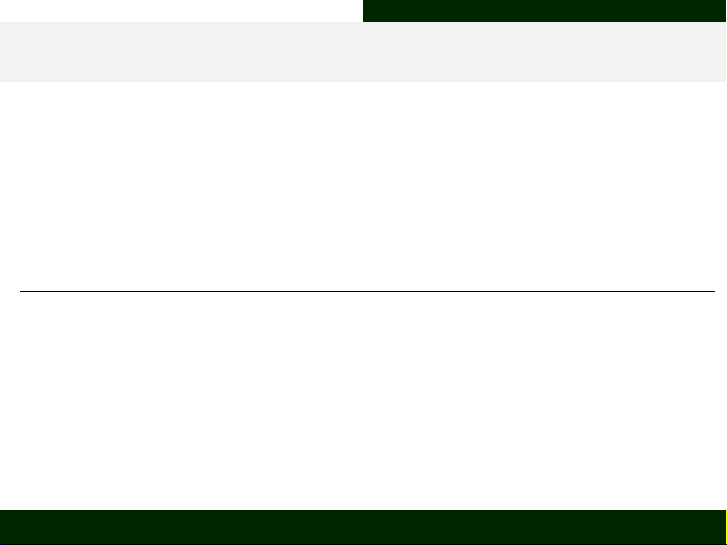Objects in R The Basics
Objects in R
R
is an
object-based
programming language hence why objects are one of the
smallest and most important units anyone attempting to handle R should be
aware of. Like words in a sentence they carry information.
Objects are characterised by attributes:
Attribute Meaning Deﬁnition
Name
R-internal name which is used to refer to the
object in question
User-deﬁned
Type R-internal class of the object in question Often user-deﬁned
Mode
R-internal class of values contained within
the object in question
Not user-deﬁned
Dimensions
Arrangement of content within the object in
question
Often user-deﬁned
Aarhus University Biostatistics - Why? What? How? 4 / 42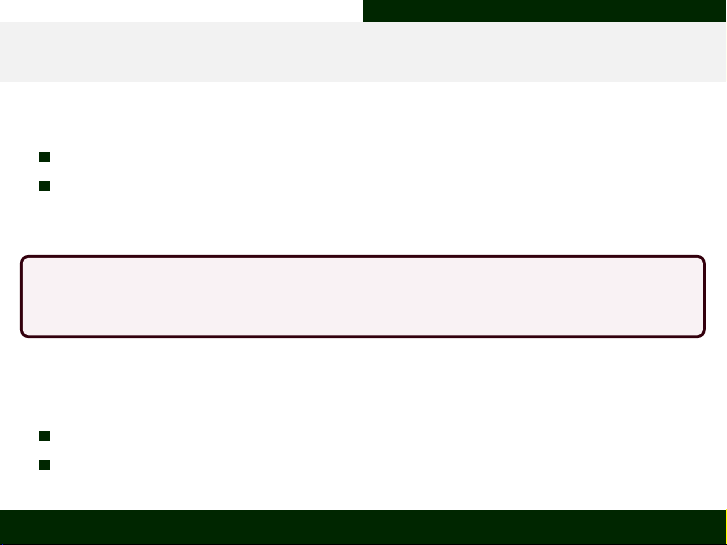Objects in R The Basics
Assigning and Removing Objects
An object is assigned some content in R as follows:
Name <- Object/Content (this is the way to go)
Name = Object/Content (avoid this)
This will add an object of the chosen name to the working environment.
Only one object of the same name can be present in the working environment at
any given time.
To
alter/remove
a speciﬁc object from your
R
working environment do either of
the following:
Name <- Object/Content (simply overwrite it)
rm(Name) (remove the object from your working environment)
Aarhus University Biostatistics - Why? What? How? 5 / 42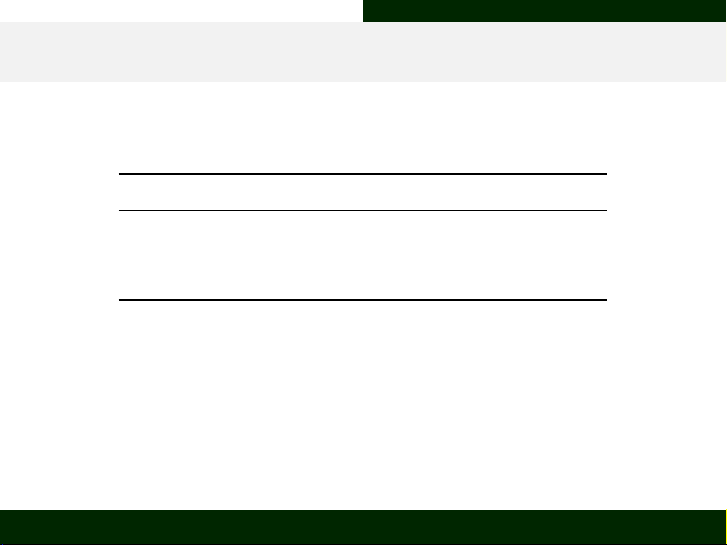Objects in R Basic R-internal Modes
Object Modes
Modes refer to the class of object-contained values. Usually, you will only
encounter some of the following basic modes:
R-internal object mode Real-world counterpart
character A letter, word or sentence
numeric A number (can have decimal points)
logical An indicator of TRUE or FALSE
Within R, the mode of any objects content can be identiﬁed using the
class()
function after having broken down the object into basic
vector
type
components.
Additionally, one may want to employ the str() function which attempts to
automatically perform some of the subsetting for you and give you an overview
of the components within your object.
Aarhus University Biostatistics - Why? What? How? 6 / 42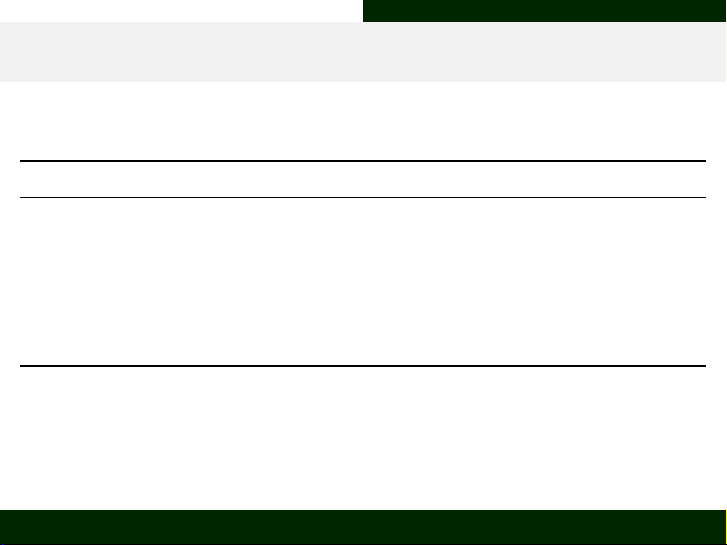Objects in R Basic R-internal Types
Object Types/Classes
Objects in R appear as different types (also referred to as classes):
R-internal object type Real-world counterpart
vector A list of variable values
factor A list of variable values with pre-deﬁned possible values
matrix A table of variable values
data frame A table of variable values
function A recipe-style functional expression detailing a process
list A list where each element corresponds to a list, table or recipe
Within R, the type of any object can be identiﬁed using the class() or
str()
function. Note that, for an object of type
vector
, the
class()
function
returns the mode of the vectors contents.
Aarhus University Biostatistics - Why? What? How? 7 / 42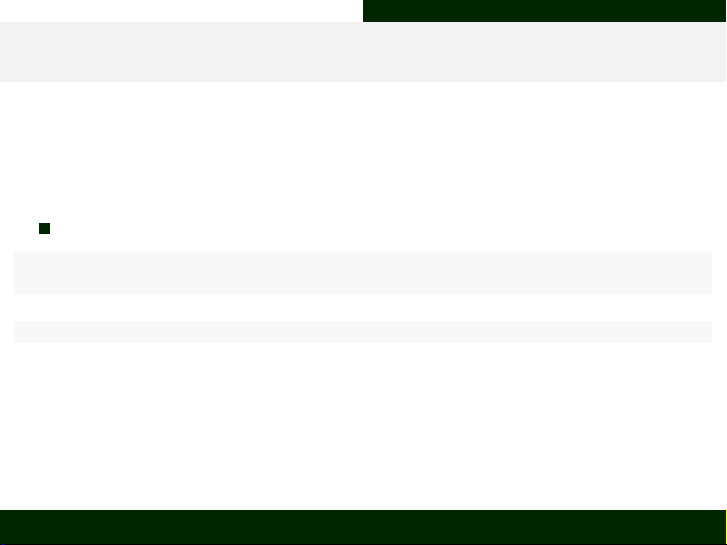Objects in R Basic R-internal Types
Vector I (character)
Vectors are created using the function c(... , ...) (“c” stands for
concatenate; “, separates individual units within the vector) in R:
A character vector:
Letters_vec <- c("A", "B", "C")
Letters_vec
##  "A" "B" "C"
class(Letters_vec)
##  "character"
You can also convert object element mode to be of character by using the
function as.character().
Aarhus University Biostatistics - Why? What? How? 8 / 42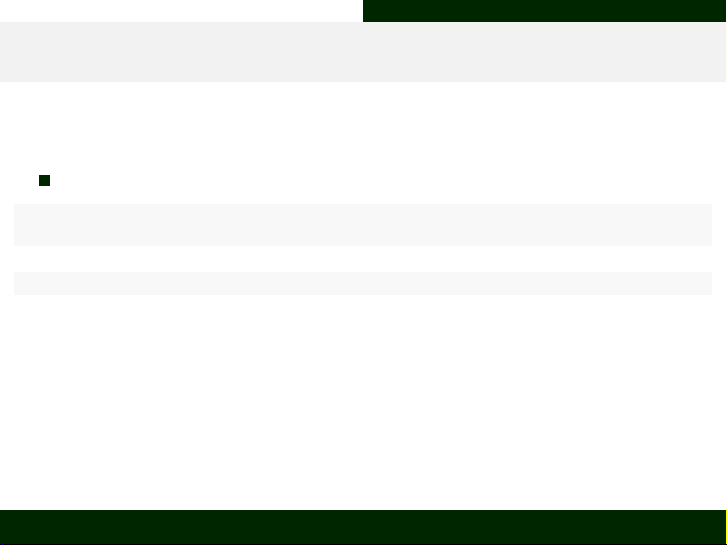Objects in R Basic R-internal Types
Vector II (numeric)
A numeric vector:
Numbers_vec <- c(1, 2, 3)
Numbers_vec
##  1 2 3
class(Numbers_vec)
##  "numeric"
You can also convert object element mode to be of numeric by using the
function as.numeric().
Aarhus University Biostatistics - Why? What? How? 9 / 42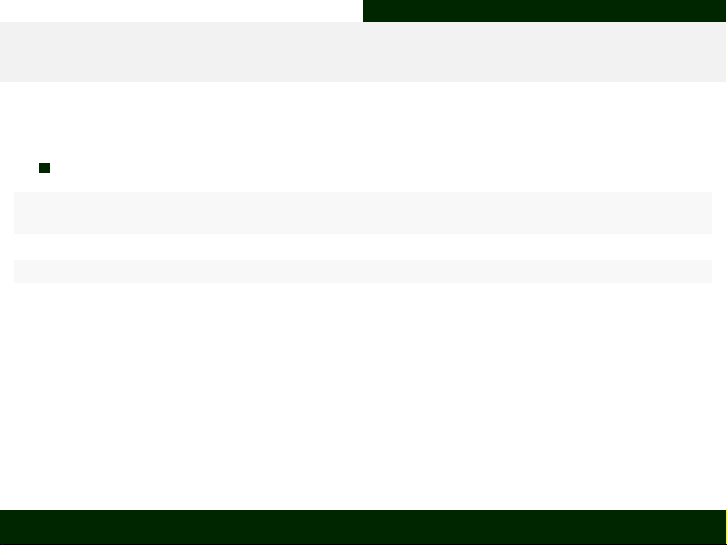Objects in R Basic R-internal Types
Vector III (logical)
A logical vector:
Logic_vec <- c(TRUE, FALSE)
Logic_vec
##  TRUE FALSE
class(Logic_vec)
##  "logical"
You can also convert object element mode to be of logical by using the
function as.logical() (keep in mind that this will only yield partially
desirable results).
Aarhus University Biostatistics - Why? What? How? 10 / 42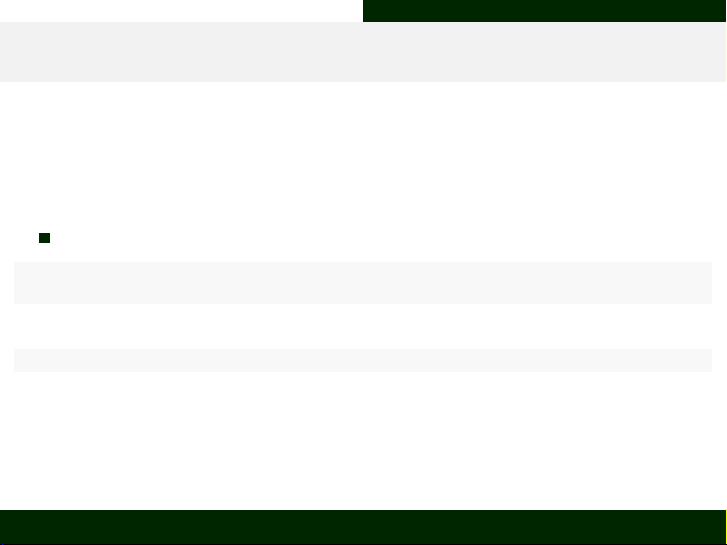Objects in R Basic R-internal Types
Factor
Factors are created using the function factor(x , levels , ...) (“x”
represents the data; “levels” indicates the preconceived levels our data should
take and is usually estimated from the data by default) in R.
A factor type object:
Letters_fac <- factor(x = c("A", "B", "C"))
Letters_fac
##  A B C
## Levels: A B C
class(Letters_fac)
##  "factor"
You can also convert object types to be of factor by using the function
as.factor().
Aarhus University Biostatistics - Why? What? How? 11 / 42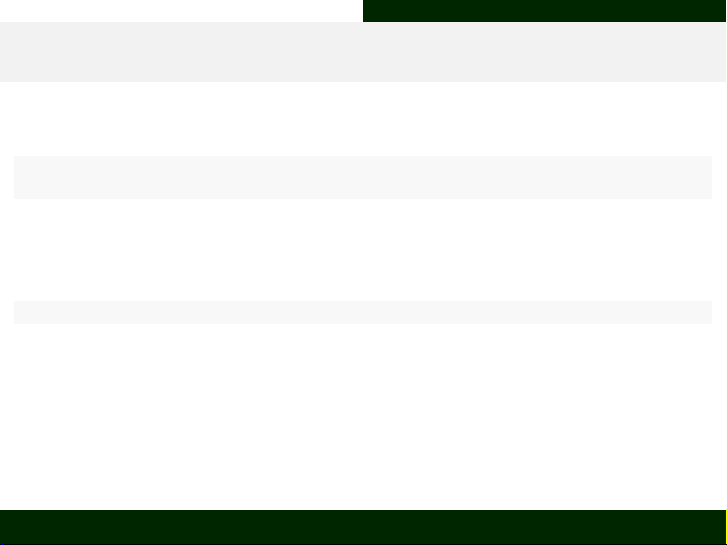Objects in R Basic R-internal Types
Lists
Lists are created using the function list(...) (“. . . represents the
objects passed to the list) in R.
Vectors_ls <- list(Numbers_vec, Letters_vec)
Vectors_ls
## []
##  1 2 3
##
## []
##  "A" "B" "C"
class(Vectors_ls)
##  "list"
Keep in mind that a list can contain every conceivable object of R within
every list position (yes, even lists of lists are possible).
You can also convert object types to be of list by using the function
as.list().
Aarhus University Biostatistics - Why? What? How? 12 / 42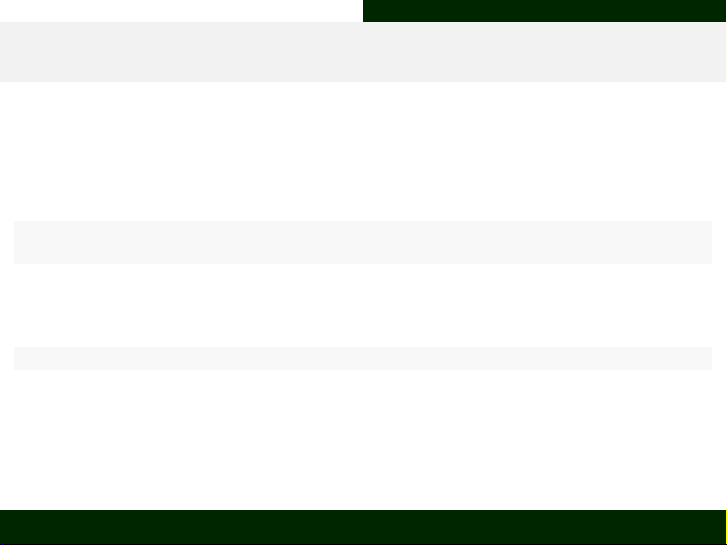Objects in R Basic R-internal Types
Matrix
Matrices are created using the function matrix(data, nrow, ncol,
byrow, dimnames) (“data” represents the data; “nrow” and “ncol” indicate
the number of rows and columns respectively, “byrow” is a logical argument
indicating whether to ﬁll the data into the matrix by row or by column,
“dimnames” let’s you ascribe names to columns and rows) in R.
Combine_mat <- matrix(data = c(Numbers_vec, Letters_vec), ncol = 2)
Combine_mat
## [,1] [,2]
## [1,] "1" "A"
## [2,] "2" "B"
## [3,] "3" "C"
class(Combine_mat)
##  "matrix"
You can also convert object types to be of matrix by using the function
as.matrix().
Aarhus University Biostatistics - Why? What? How? 13 / 42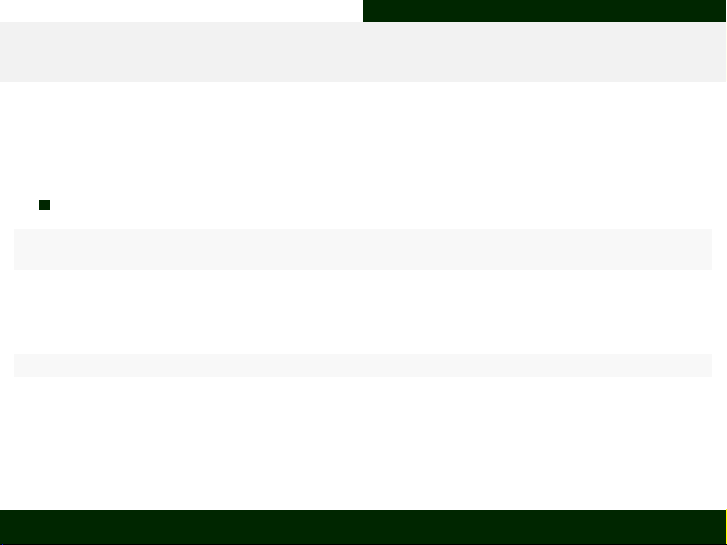Objects in R Basic R-internal Types
Data Frame I (creating from a matrix)
Data Frames are created using the function data.frame(...) (“. . . can
stand for a matrix or index individual columns) in R.
Creating a data.frame from a matrix:
Combine_df <- data.frame(Combine_mat)
Combine_df
## X1 X2
## 1 1 A
## 2 2 B
## 3 3 C
class(Combine_df)
##  "data.frame"
You can also edit the names of columns and rows in a data.frame object
using the commands colnames() and rownames() respectively.
Aarhus University Biostatistics - Why? What? How? 14 / 42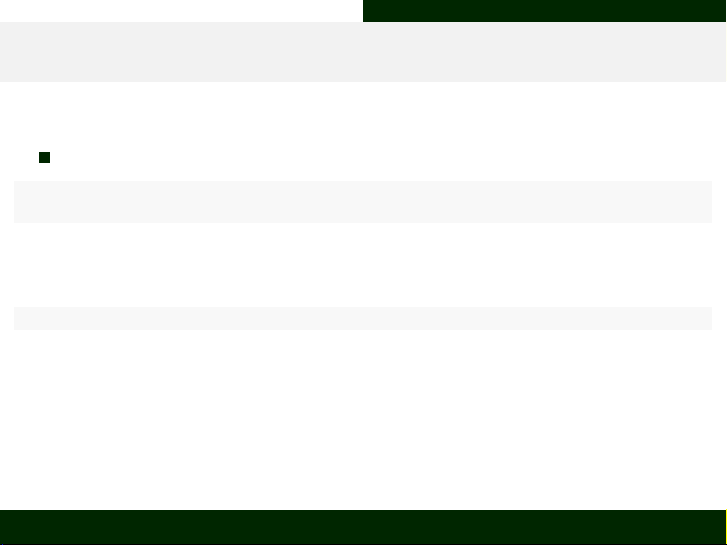Objects in R Basic R-internal Types
Data Frame II (creating with individual columns)
Creating a data.frame from vectors as individual columns:
Combine2_df <- data.frame(Numbers = Numbers_vec, Letters = Letters_vec)
Combine2_df
## Numbers Letters
## 1 1 A
## 2 2 B
## 3 3 C
class(Combine_df)
##  "data.frame"
You can also convert object types to be of data.frame by using the function
as.data.frame().
Aarhus University Biostatistics - Why? What? How? 15 / 42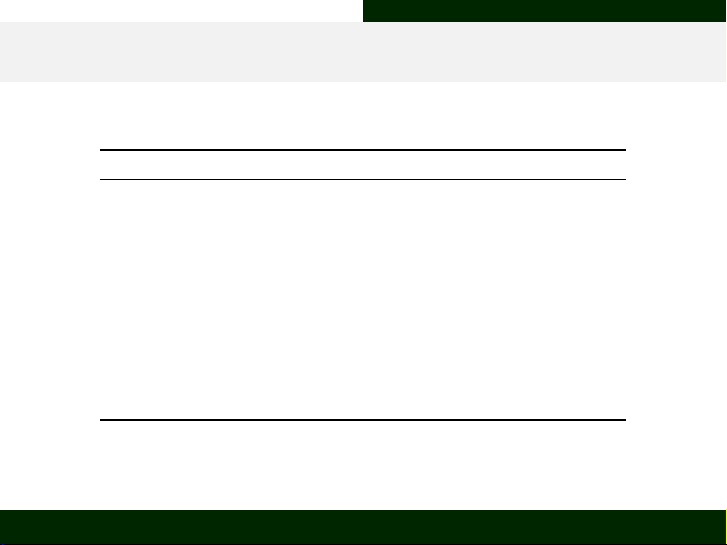Objects in R CheatSheet Overview
Converting Modes
Target Mode Function What Happens
numeric as.numeric FALSE > 0
TRUE > 1
"1", "2", "..." > 1, 2, ...
"A" > NA
character as.character 1, 2, ... > "1", "2", "..."
FALSE > "FALSE"
TRUE > "TRUE"
logical as.logical 0 > FALSE
any number that isn’t 0 > TRUE
"FALSE"/"F" > FALSE
"TRUE"/"T" > TRUE
any character that isn’t any of the above > NA
Aarhus University Biostatistics - Why? What? How? 16 / 42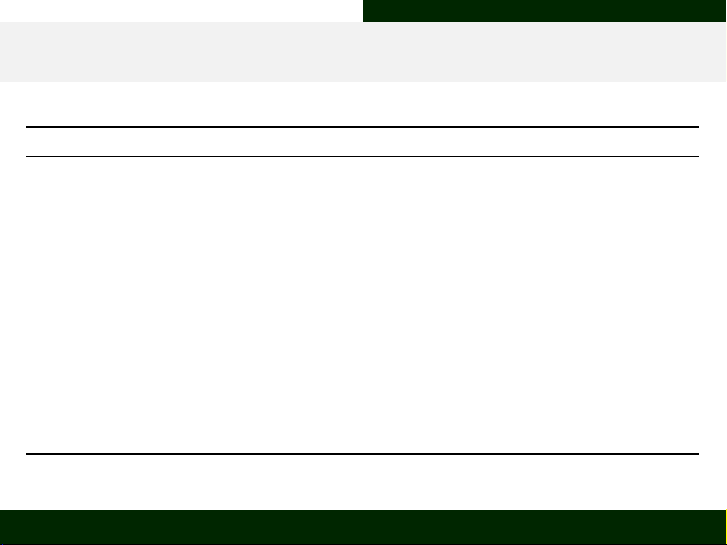Objects in R CheatSheet Overview
Converting Types
Target Type Function What Happens
vector as.vector factor levels are dropped
matrices are made into vectors one column at a time
data frames are made into vectors but remain in an array arrangement
lists remain as lists
factor as.factor factor levels are established from vector values
factors levels drawn from a column-wise vectorisation of matrices
does not work on data frames or lists
matrix as.matrix Puts content of vectors or factor into a single column
data frames remain unaltered as far as data structure goes
lists turn into cells with type and number of items of list positions
data frame as.data.frame each object gets put into a separate column
matrices remain unaltered as far as data structure goes
every list position is made into a column
list as.list every element gets put into an individual list position
columns of data frames occupy list positions
Aarhus University Biostatistics - Why? What? How? 17 / 42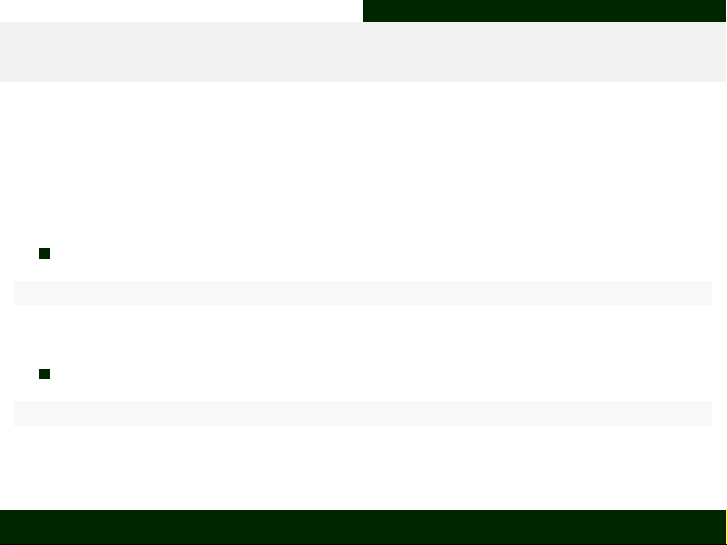Objects in R Inspecting and Subsetting Objects in R
Object Dimensions (matrices and data.frames)
Object dimensions are the last of the essential attributes of objects we will
consider. They tell you how data is arranged. They are assessed using the
dim(...) function in R (“. . . stands for any given object name in R):
Matrices have dimensions of rowcount x columncount
dim(Combine_mat)
##  3 2
Data Frames also have dimensions of rowcount x columncount
dim(Combine_df)
##  3 2
Aarhus University Biostatistics - Why? What? How? 18 / 42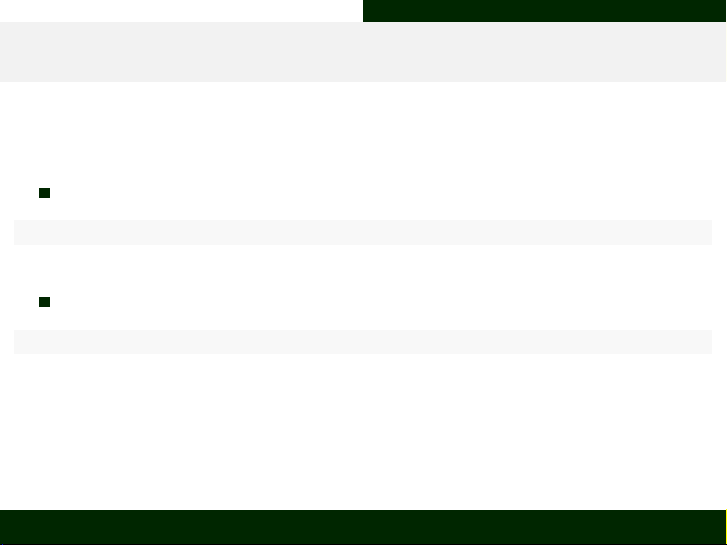Objects in R Inspecting and Subsetting Objects in R
Object Dimensions (vectors, factors, lists)
Vectors, Factors and Lists don’t have dimensions. . .
c(dim(Letters_vec), dim(Letters_fac), dim(Vectors_ls))
## NULL
. . . they only have a length
c(length(Letters_vec), length(Letters_fac), length(Vectors_ls))
##  3 3 2
Aarhus University Biostatistics - Why? What? How? 19 / 42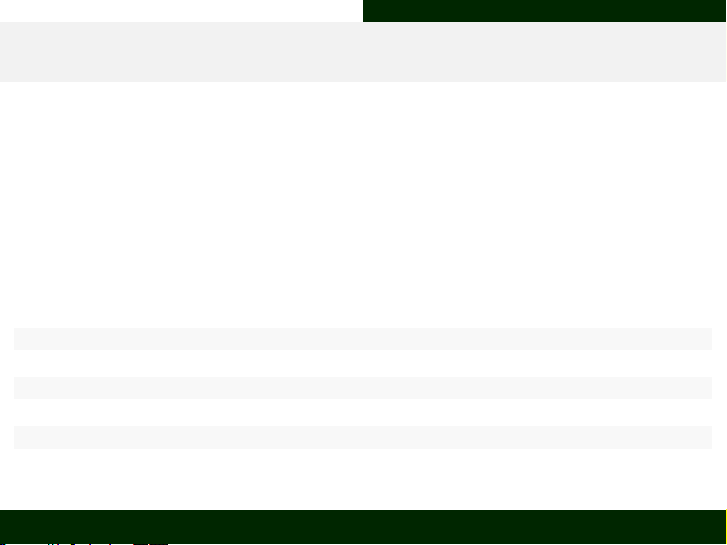Objects in R Inspecting and Subsetting Objects in R
The Naming System (data.frames)
Names can serve as labels to elements of an object and are implemented/
assigned using the names() function at the most basic level (i.e. “for
vectors
and
factors
”). Further implementations of names come in the form
of the following functions:
- colnames() (column labels, data.frames)
- rownames() (row labels, data.frames)
- dimnames() (dimension labels, matrices)
colnames(Combine2_df)
##  "Numbers" "Letters"
rownames(Combine2_df)
##  "1" "2" "3"
Combine2_df\$Numbers # subsetting by column 'Numbers'
##  1 2 3
Aarhus University Biostatistics - Why? What? How? 20 / 42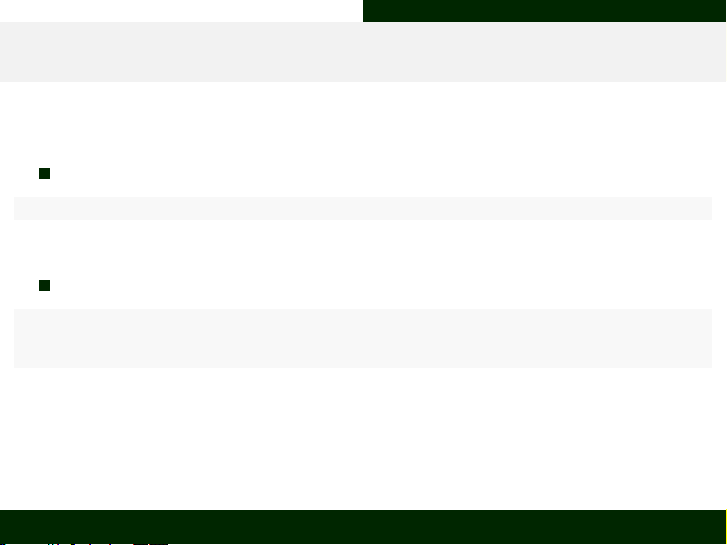Objects in R Inspecting and Subsetting Objects in R
The Naming System (vectors)
Our vectors don’t have names assigned yet:
names(Numbers_vec)
## NULL
Let’s do that:
SubNames1 <- Numbers_vec
names(SubNames1) <- Letters_vec
SubNames1
## A B C
## 1 2 3
Aarhus University Biostatistics - Why? What? How? 21 / 42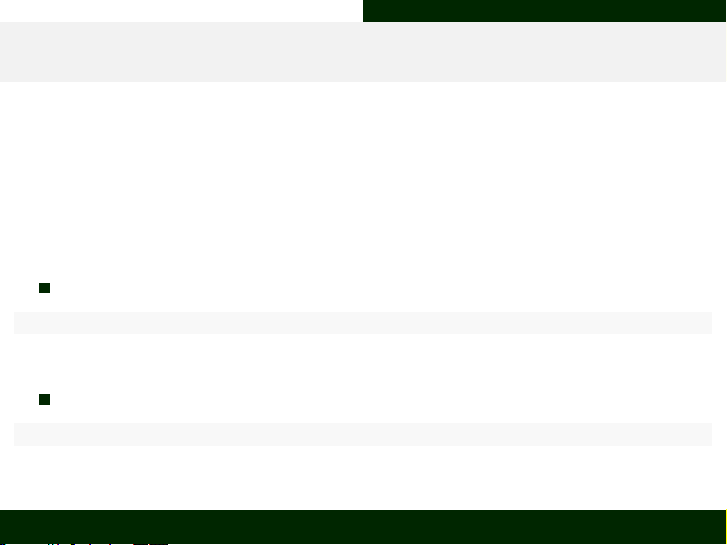Objects in R Inspecting and Subsetting Objects in R
The Indexing System (matrices and data.frames)
The indexing system is basically the numerical counterpart to the naming
system and called into action by the use of square brackets ([]):
- [elementnumber] for vectors and factors
- [[elementnumber]] for lists
- [rownumber, columnnumber] for data.frames and matrices
First element of second column of our matrix:
Combine_mat[1, 2]
##  "A"
Entire ﬁrst column of our data frame:
Combine_df[, 1]
##  1 2 3
## Levels: 1 2 3
Aarhus University Biostatistics - Why? What? How? 22 / 42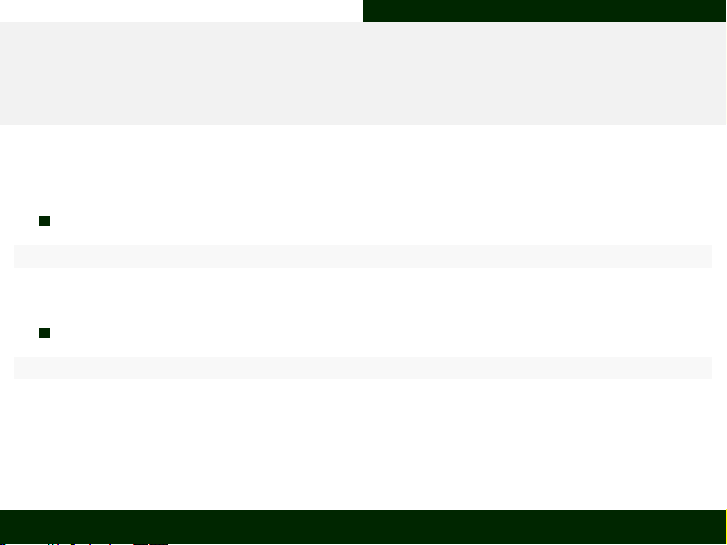Objects in R Inspecting and Subsetting Objects in R
The Indexing System (vectors, factors and
lists)
Third element of our letter vector:
Letters_vec
##  "C"
First element of our list:
Vectors_ls[]
##  1 2 3
Aarhus University Biostatistics - Why? What? How? 23 / 42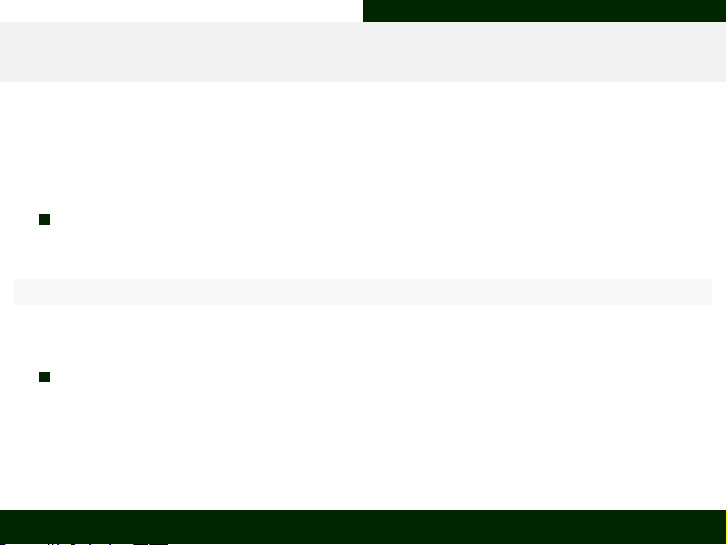Functionality in R Vectorisation
What is Vectorisation and why should I care?
R is a vectorised language.
What does that mean?
Any mathematical operation is applied to every element in an object:
Numbers_vec + 1
##  2 3 4
Why care?
Because it greatly inﬂuences how you do calculations in R.
Aarhus University Biostatistics - Why? What? How? 25 / 42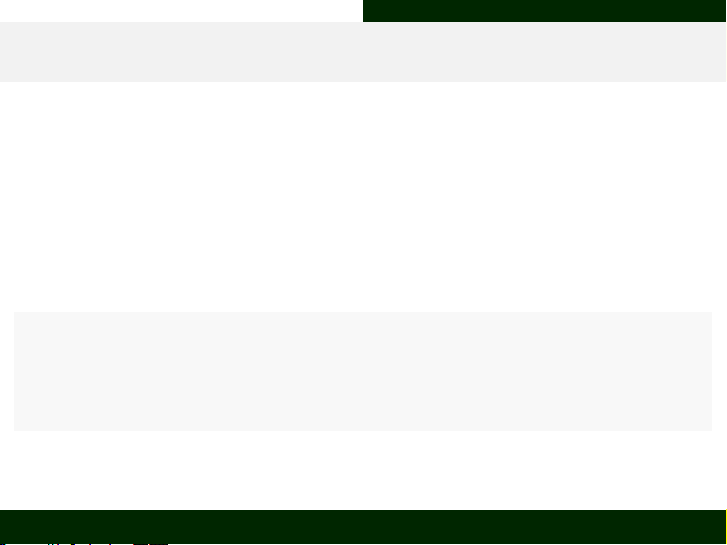Functionality in R User-deﬁned Functions
Writing Functions in R
Functions are special objects within R as they carry information for
processing objects. Functions require the following components:
- Name - each function has a name
- Argument(s) - give additional information to the function
- Call - a function needs to be called to exert its effect
Users can create their own functions in R as follows:
Plus1 <- function(x) {
# function has an argument of `x`
y <- x + 1 # 1 is added to the object `x` and saved as `y`
return(y) # object `y` is returned
}
Plus1(Numbers_vec) # call the function on thes Numbers vector
##  2 3 4
# indicate comments in the code - these are not run but can help explain stuff to the user
Aarhus University Biostatistics - Why? What? How? 26 / 42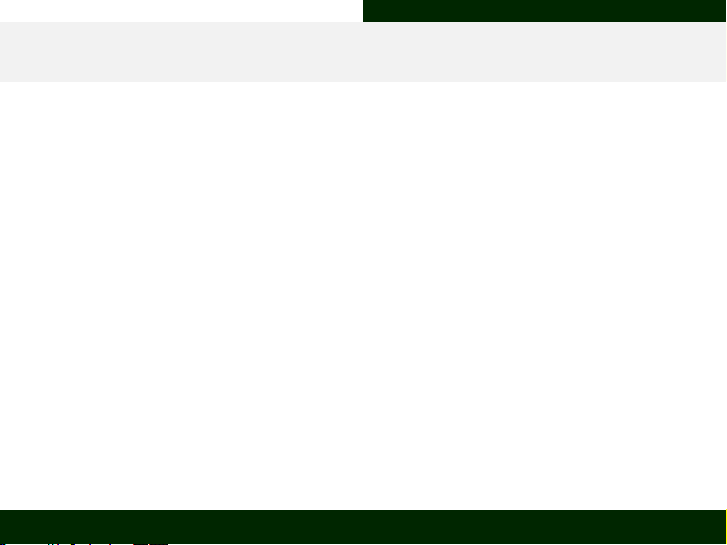Functionality in R Packages
What are R packages?
Packages are Rs way of supplying the user with a widened range of
functionality (just like a mod to a computer game or bonus tracks on a CD).
There are thousands of packges for R which have been designed by other R
users, tested vigorously, and are available freely for you to use.
All packages are available via the Comprehensive R Archive Network
(https://cran.r-project.org/) and an overview of available packages can be
retrieved here:
https://cran.r-project.org/web/packages/available_packages_by_date.html.
Aarhus University Biostatistics - Why? What? How? 27 / 42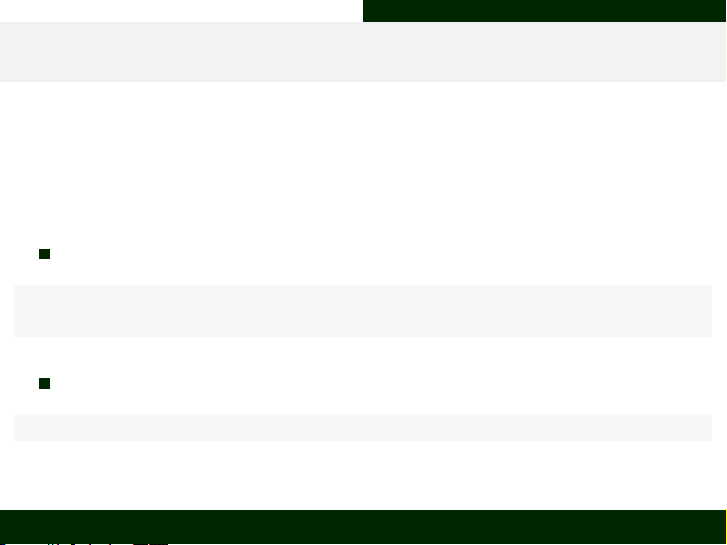Functionality in R Packages
What do I do with packages?
You install and load them into R!
This is done in a two-step process:
install.packages() is used to install packages in R
# vegan is a common library of functionality in biostatistics
install.packages("vegan")
library() is used to load them into the current working environment
library(vegan)
Aarhus University Biostatistics - Why? What? How? 28 / 42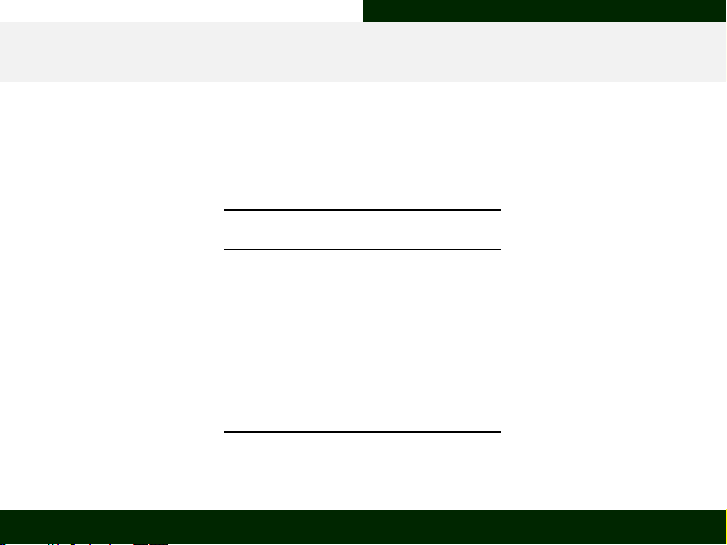Functionality in R Statements
Logical statements
Logical statements are indicators of whether something is true or not. We
use those frequently in real life (i.e. ‘Is a 10 ECTS course worth more to me
than a 5 ECTS course?’). R implements these with the following operators:
Operator Translation
== “equals”
!= “does not equal”
< “is smaller”
<= “is equal or smaller”
> “is bigger”
>= “is equal or bigger”
These statements return an element of mode logical (TRUE or FALSE).
Aarhus University Biostatistics - Why? What? How? 29 / 42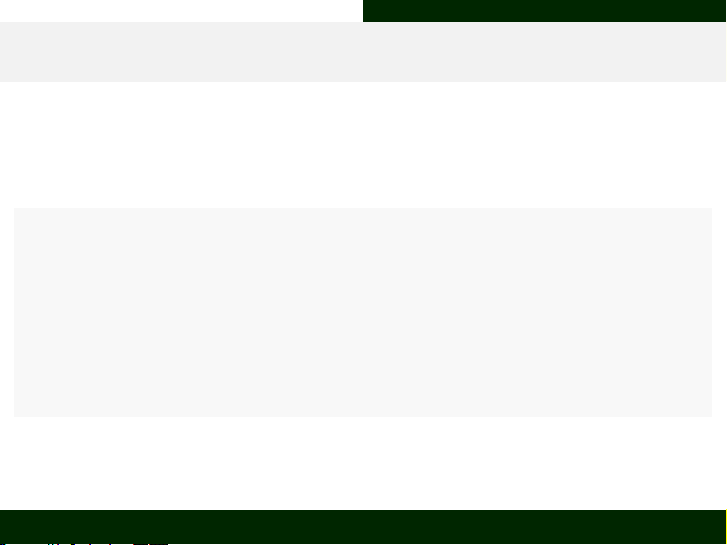Functionality in R Statements
if() statements
if() statements build on logical statements. They test whether something
is correct and then act on it:
# is 1 smaller than 2?
if (Numbers_vec < Numbers_vec) {
# if the statement is correct
print("Is smaller") # print this to the console
} else {
# if the statement is not correct
print("Is not smaller") # print this to the console
}
##  "Is smaller"
Aarhus University Biostatistics - Why? What? How? 30 / 42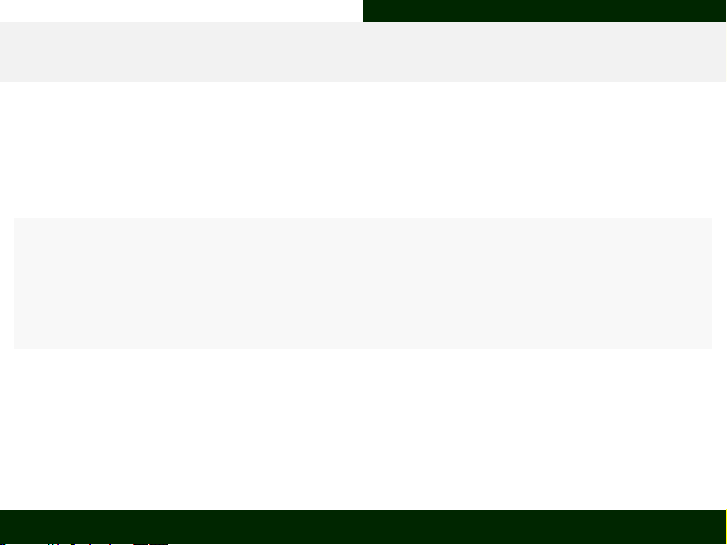Functionality in R Loops
for() loops
for() loops are in action whilst an indiciator is within a speciﬁed data
range:
# loop from one to length of the letter vector in steps of 1
for (i in 1:length(Letters_vec)) {
# print current itteration element of letters vector
print(Letters_vec[i])
}
##  "A"
##  "B"
##  "C"
Aarhus University Biostatistics - Why? What? How? 31 / 42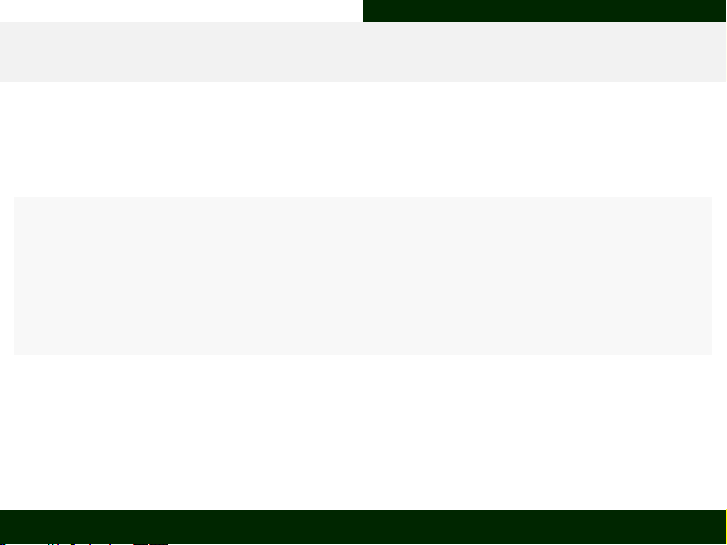Functionality in R Loops
while() loops
while() loops work a lot like for() loops and are in action whilst an
indiciator statement is TRUE:
# while our data frame has equal to or less than 3 columns
while (dim(Combine_df) <= 3) {
# bind letters factor vector to data frame as column
Combine_df <- cbind(Combine_df, Letters_fac)
}
Combine_df # inspect the result
## X1 X2 Letters_fac Letters_fac
## 1 1 A A A
## 2 2 B B B
## 3 3 C C C
Aarhus University Biostatistics - Why? What? How? 32 / 42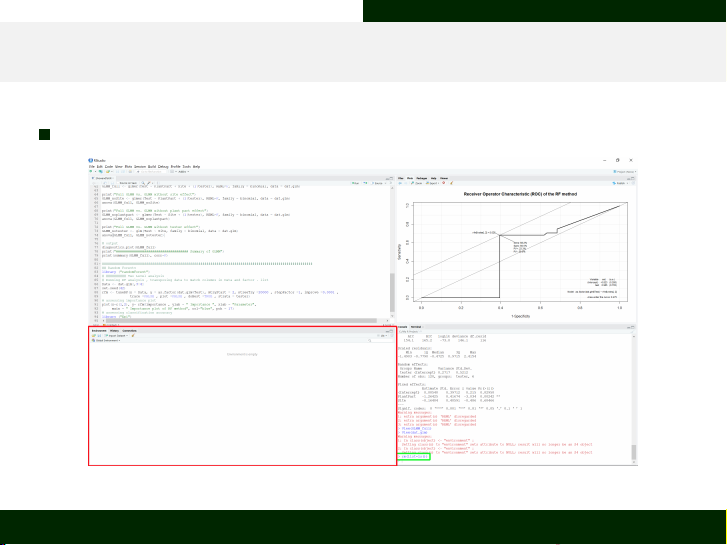Functionality in R Useful Commands In R
rm(list=ls()) I
rm(list=ls()) clears the entire working environment
Aarhus University Biostatistics - Why? What? How? 33 / 42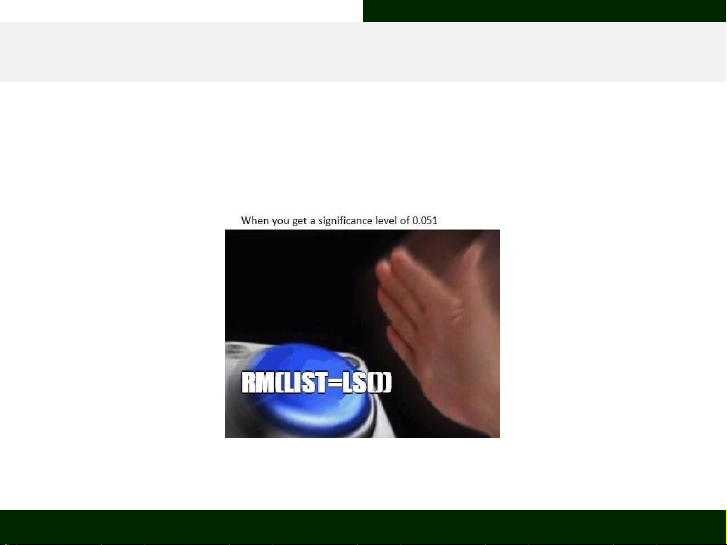Functionality in R Useful Commands In R
rm(list=ls()) II
rm(list=ls()) is extremely useful and one should use this before
sourcing (running an entire script) any R document to avoid inﬂuence of
remnants of previous R sessions on the current analysis.
Simply code this to be your ﬁrst line!
Aarhus University Biostatistics - Why? What? How? 34 / 42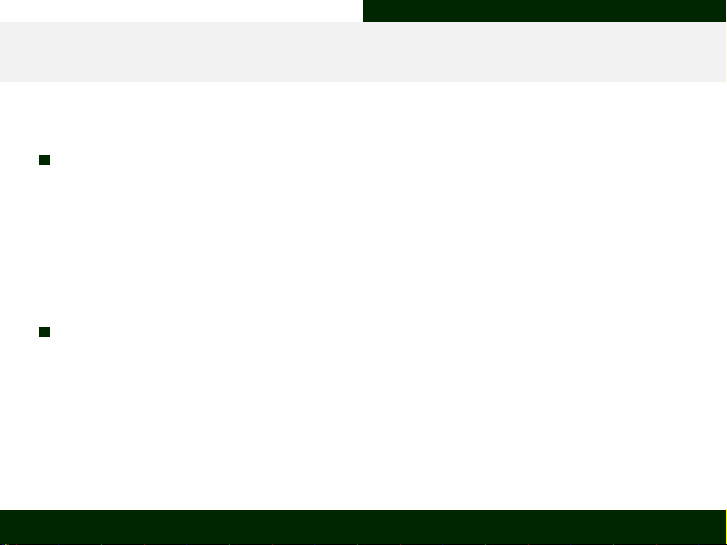Functionality in R Useful Commands In R
getwd() and setwd()
getwd() returns the current working directory
can identify the project folder if coded into script fairly early (usually second
line)
can be used to soft code the working directory
setwd() is used to set speciﬁc working directories
can be used to address speciﬁc folders on your hard drive as the current
working directory
often used to hard code the working directory (please avoid this at all cost!)
Aarhus University Biostatistics - Why? What? How? 35 / 42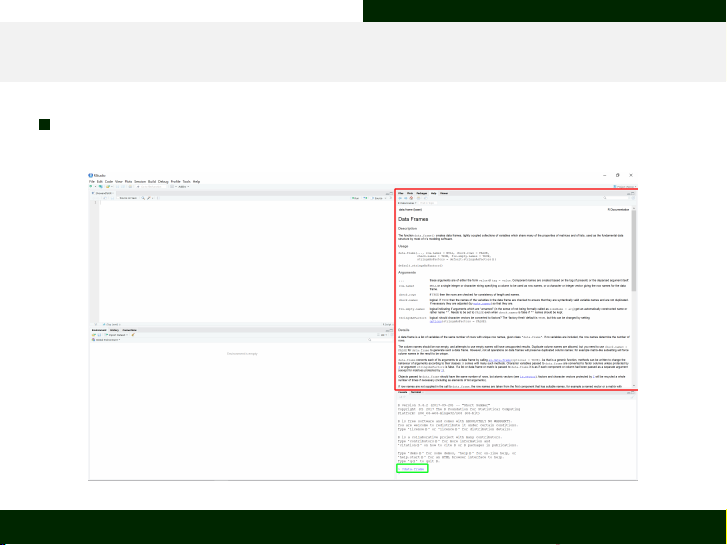Functionality in R Useful Commands In R
?
? followed by a function name, this will open the help documentation for
said function
Aarhus University Biostatistics - Why? What? How? 36 / 42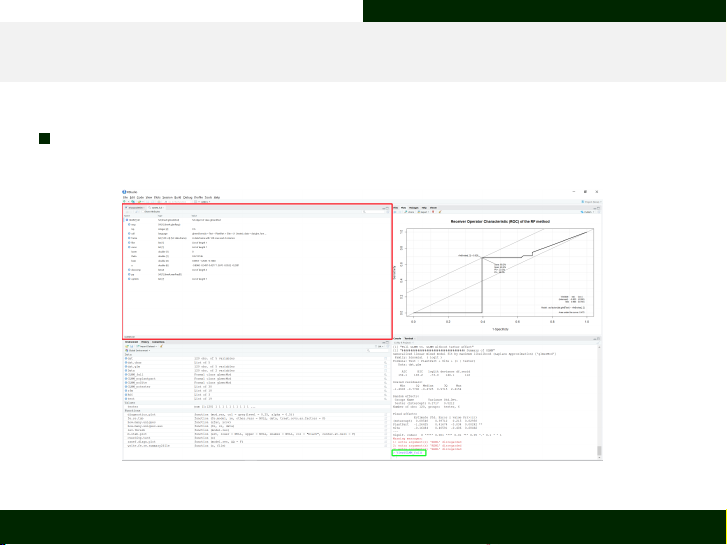Functionality in R Useful Commands In R
View()
View() is a very neat command that lets the user inspect any object of
their choosing in a separate tab
Aarhus University Biostatistics - Why? What? How? 37 / 42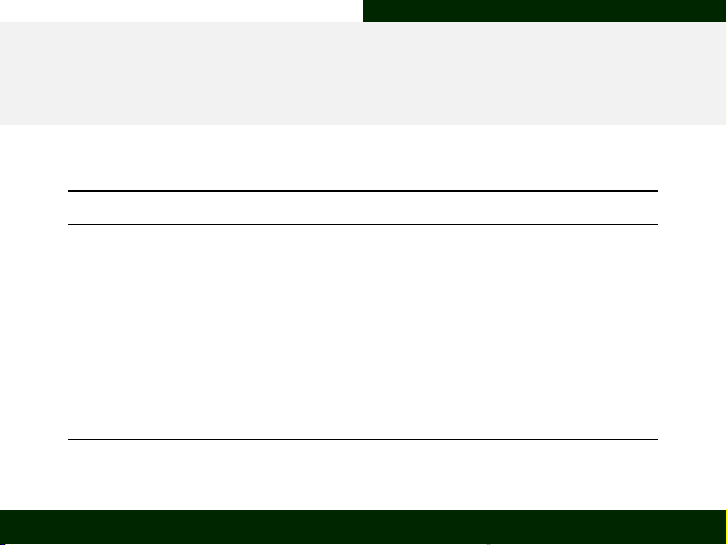Exercise
Creating and Inspecting Objects (vectors and
factors)
Create the following vectors or factors:
Name Content
Letters_vec A vector reading “A”, “B”, “C”
Numbers_vec A vector reading 1, 2, 3
Logic_vec A vector reading TRUE, FALSE
Big_vec A vector of the elements of the ﬁrst three vectors
Seq_vec A vector reading as a sequence of full numbers from 1 to 20
Letters_fac A factor reading “A”, “B”, “C”
Numbers_fac A factor reading 1, 2, 3
Constrained_fac A factor reading 1, 2, 3, levels 1 and 2 are allowed
Expanded_fac A factor reading 1, 2, 3 levels 1 - 4 are allowed
Inspect these objects for their classes and dimensions!
Aarhus University Biostatistics - Why? What? How? 39 / 42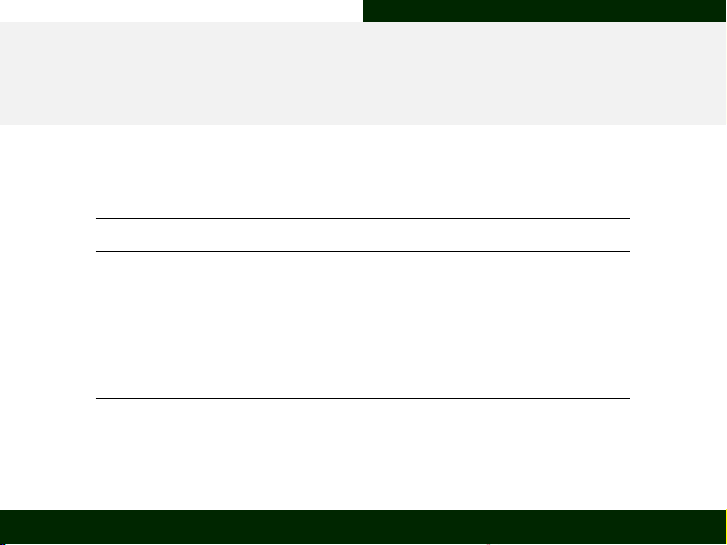Exercise
Creating and Inspecting Objects (matrices,
data.frames and lists)
Create the following objects:
Name Content
Combine_mat Numbers_vec and Letters_vec in columns of a matrix
Pivot_mat First two vectors in distinct rows of a matrix
Names_mat The above matrix with meaningful names
Combine_df The ﬁrst matrix we established as a data frame
Names_df The previous data frame with meaningful names
Vectors_ls The ﬁrst two vectors we created as a list
Inspect these objects for their classes and dimensions!
Aarhus University Biostatistics - Why? What? How? 40 / 42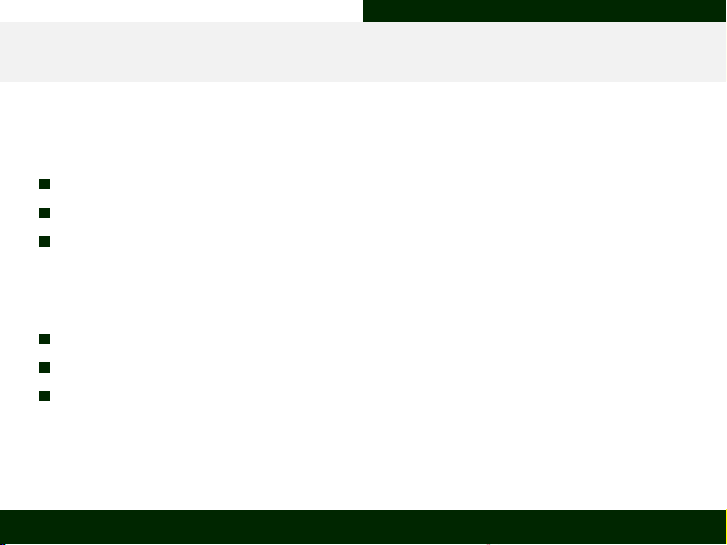Exercise
Statements and Loops
Test the following statements:
Numbers_vec contains more elements than Letters_fac
The ﬁrst column of Combine_df is shorter than Vectors_ls
The elements of Letters_vec are the same as the elements of
Letters_fac
Write the following loops:
Print each element of Vectors_ls
Print each element of Numbers_vec + 1
Subtract 1 from each element of the ﬁrst column of Combine_mat and
print each element separately
Aarhus University Biostatistics - Why? What? How? 41 / 42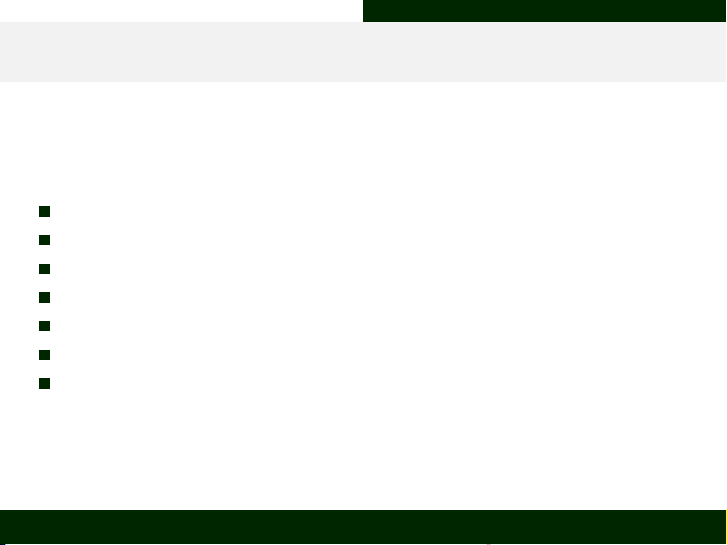Exercise
Using the Useful Commands
Do the following: